# Test: Stoichiometry and Stoichiometric Calculations

## 20 Questions MCQ Test NCERTs for NEET: Textbooks, Tests & Solutions | Test: Stoichiometry and Stoichiometric Calculations

Description
Attempt Test: Stoichiometry and Stoichiometric Calculations | 20 questions in 20 minutes | Mock test for NEET preparation | Free important questions MCQ to study NCERTs for NEET: Textbooks, Tests & Solutions for NEET Exam | Download free PDF with solutions
QUESTION: 1

### 1 g of Mg is burnt in a closed vessel containing 0.5 g of O2. Which reactant is limiting reagent and how much of the excess reactant will be left?

Solution: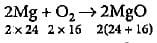48 g of Mg requires 32 g of O2
1 g of Mg requires 32/48 = 0.66 g of O2
Oxygen available = 0.5 g
Hence, O2 is limiting reagent.
32 g of O2 reacts with 48 g of Mg
0.5 g of O2 will react with 48/32 x 0.5 = 0.75 g of Mg
Excess of Mg = (1.0 - 0.75) = 0.25 g

QUESTION: 2

### In a reaction container, 100 g of hydrogen and 100 g of CI2 are mixed for the formation of HCl gas. What is the limiting reagent and how much HCl is formed in the reaction?

Solution: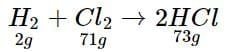2 g of H2 reacts with 71 g of CI2
100 g of H2 will react with 71/2 x 100 = 3550g of CI2
Hence, CI2 is the limiting reagent.
71 g of CI2 produces 73 g of HCl
100g of CI2 will produce 73/71 x 100 = 102.8 g of HCl

QUESTION: 3

### If 40 g of CaCO3 is treated with 40 g of HCl, which of the reactants will act as limiting reagent?

Solution: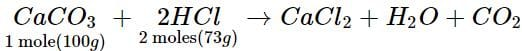100 g of CaCO3 reacts with 73 g of HCl
40 g of CaCO3 will react with 73/100 x 40 = 29.2 g of HCl
Since CaCO3 is completely consumed and some amount (40 - 29.2 = 10.8g) of HCl remains unreacted and hence, CaCO3 is limiting reagent.

QUESTION: 4

The weight of AgCl precipitated when a solution containing 5.85 g of NaCl is added to a solution containing 3.4 g of AgNO3 is

Solution:

AgNO3 + NaCl → AgCl + NaNO3
No. of moles of AgNO3 = 3.4/170 = 0.02
No, of moles of NaCl = 5.85/58.5 = 0.1
Limiting reagent = AgNO3
1 mole of AgNO3 produces 1 mole of AgCl
0.02 mole of AgNO3 will produce 0.02 mole of AgCl
Weight of AgCl produced = 0.02 x 143.5 = 2.870 g.

QUESTION: 5

How much oxygen is required for complete combustion of 560 g of ethene?

Solution: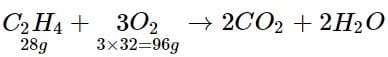28 g of C2H4 requires 96 g of O2
560 g of C2H4 requires 96/28 x 560
= 1920 g or 1.92 kg of O2

QUESTION: 6

What is the mass percent of oxygen in ethanol?

Solution:

Molecular formula of ethanol = C2H5OH
Molar mass of ethanol of 2 x 12.01 + 6 x 1.008 + 16 = 46.068g
Mass percent of oxygen = 16/46.068 x 100 = 34.73%

QUESTION: 7

How much mass of sodium acetate is required to make 250 mL of 0.575 molar aqueous solution?

Solution:

Molar mass of sodium acetate (CH3COONa)
= 82.0245 g/mol
Mass of CH3COONa required to make 250 mL of 0.575 M solution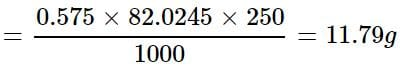QUESTION: 8

How much copper is present in 50 g of CuSO4?

Solution:

Molar mass of CuSO4 = 63.5 + 32 + 4 x 16 = 159.5 g
Mass of copper present in 159.5 g of CuSO4 = 63.5 g
∴ Mass of copper present in 50 g of CuSO4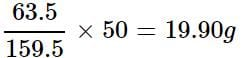QUESTION: 9

A solution is prepared by adding 5 g of a solute 'X' to 45 g of solvent 'Y'. What is the mass per cent of the solute 'X'?

Solution:

Mass percent of X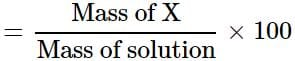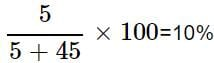QUESTION: 10

An impure sample of silver (1.5 g) is heated with S to form 0.124 g of Ag2S. What was the per cent yield of Ag2S?

Solution: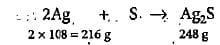216 g of Ag forms 248 g of Ag2S
1.5 g of Ag forms 248/216 x 1.5 = 1.722 g of Ag2S
% yield of Ag2S = 0.124/1.722 x 100 = 7.2%

QUESTION: 11

2.82 g of glucose is dissolved in 30 g of water. The mole fraction of glucose in the solution is

Solution:

No. of moles of glucose = 2.82/180 = 0.01567
No. of moles of water = 30/18 = 1.667
Total no. of moles of solution = 0.01567 + 1.667 = 1.683
Mole fraction of glucose = 0.01567/1.683 = 0.0093 = 0.01

QUESTION: 12

What volume of water is to be added to 100 cm3 of 0.5 M NaOH solution to make it 0.1 M solution?

Solution:

M1V1 = M2V2
0.5 x 100 = 0.1 x V2
V2 = 500 cm3
Volume of water to be added to 100 cm3 of solution
= 500 - 100 = 400 cm3

QUESTION: 13

The final molarity of a solution made by mixing 50 mL of 0.5 M HCl, 150 mL of 0.25 M HCl and water to make the volume 250 mL is

Solution:

M1V= M2V= MV
0.5 x 50 + 0.25 x 150 = M x 250
M = (25 + 37.5)/250 = 0.25 M

QUESTION: 14

A solution is made by dissolving 49 g of H2SO4 in 250 mL of water. The molarity of the solution prepared is

Solution:

Molarity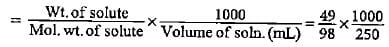= 2 M

QUESTION: 15

What is the concentration of copper sulphate (in mol L-1) if 80 g of it is dissolved in enough water to make a final volume of 3 L?

Solution:

Molar mass of CUSO4 = 63.5 + 32 + 64 = 159.5
Moles of CUSO4 = 80/159.5 = O.50
Volume of solution = 3 L= 0.167 mol L-1

QUESTION: 16

4.28 g of NaOH is dissolved inwater and the solution is made to 250 cc. What will be the molarity of the solution?

Solution:

No. of moles of NaOH = 4.28/40 = 0.107 Volume of solution = 250 cm3
M = n/V(in L) = 0.107/250 x 1000 = 0.428 mol L-1

QUESTION: 17

What volume of 5 M Na2SO4 must be added to 25 mL of 1 M BaCl2 to produce 10 g of BaSO4?

Solution:

Na2SO4 + BaCI2 → BaSO4 + 2NaCl
No. of moles of BaSO4 = w/M = 10/233 = 0.0429
∴ No. of moles of Na2SO4 needed = M x V/1000
Or 0.0429 = 5 x V/1000
V = 8.58 mL

QUESTION: 18

What will be the molarity of the solution in which 0.365 g of HCl gas is dissolved in 100 mL of solution?

Solution:

No. of moles in 0.365 g of HCl = 0.365/36.5 = 0.01
Volume of solution in L = 100/1000 = 0.1 L
Molarity = n/V (in L) = 0.01/0.1 = 0.1 M

QUESTION: 19

What will be the molality of the solution made by dissolving 10 g of NaOH in 100 g of water?

Solution:

Molality =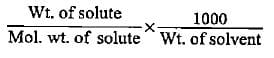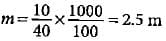QUESTION: 20

What will be the molality of chloroform in the water sample which contains 15 ppm chloroform by mass?

Solution:

15 ppm = 15/106 x 102 = 1.5 x 10-3g
Molality of CHCl3 solution = 1.5 x 10-3/100 x 1000/119.5
= 1.25 x 10-4 mUse Code STAYHOME200 and get INR 200 additional OFF Use Coupon Code